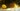# Javascript 中的数字截断(Number Truncation)

``````const number = 80.6;
// Old Way
function truncNumber() {
number < 0 ? Math.ceil(number) : Math.floor(number);
}
truncNumber(number); // 80

// ✅ES6 Way
const es6 = Math.trunc(number); // 80``````

## 例子

`Math.trunc()` 简单地截断(剪除)数字小数点和小数点后面的部分。无论参数是正数还是负数。

``````Math.trunc(80.9); // 80
Math.trunc(80.8); // 80
Math.trunc(80.8); // 80
Math.trunc(80.6); // 80
Math.trunc(80.5); // 80
Math.trunc(80.4); // 80
Math.trunc(80.3); // 80
Math.trunc(80.2); // 80
Math.trunc(80.1); // 80
Math.trunc(-80.1); // -80``````

``````Math.trunc('80.1'); // 80
Math.trunc('hello'); // NaN
Math.trunc(NaN); // NaN
Math.trunc(undefined); // NaN
Math.trunc(); // NaN``````

## 使用 parseInt 进行数字截断

``````parseInt(80.1); // 80
parseInt(-80.1); // -80
parseInt('80.1'); // 80
parseInt('hello'); // NaN
parseInt(undefined); // NaN
parseInt(); // NaN``````

## Math.trunc() 与 parseInt() 相比

`parseInt` 主要用于数字参数。所以如果你正在处理数字，`Math.trunc()` 是更好的方法。

jsPerf(英文): Math.trunc() 与 parseInt() 相比

## 使用 parseInt 的缺陷

``````const number = 1000000000000000000000.5;
const result = parseInt(number);
console.log(result); // 1 <-- 😱``````

☝️ 为什么会这样呢？因为我们的参数不是一个 `string`，于是 `parseInt` 首先将这个参数转化为一个 `string`

``````const number = 1000000000000000000000.5;
const result = number.toString();
console.log(result); // "1e+21"``````

## 浏览器兼容性

MDN：Math.trunc 的浏览器兼容性

`Math.trunc` 浏览器填充(Polyfill)，在不支持的浏览器中提供服务：

``````if (!Math.trunc) {
Math.trunc = function (v) {
v = +v;
if (!isFinite(v)) return v;

return v - (v % 1) || (v < 0 ? -0 : v === 0 ? v : 0);
};
}``````

``````if (!Math.trunc) {
Math.trunc = function (v) {
v = +v;
return v - (v % 1) || (!isFinite(v) || v === 0 ? v : v < 0 ? -0 : 0);
};
}``````

## 社区支持

### 位操作符解决方法

``console.log(~~80.6); // 80``

### 按位或(OR) `|`

``console.log(80.6 | 0); // 80``

### toFixed 解决方法

``(80.645).toFixed(); // “80”``

``Number((80.645).toFixed()); // 80``

``Number((80.645).toFixed(1)); // 80.6``